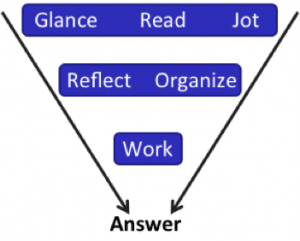# Tackling Multishape Geometry on the GMAT

by , Dec 6, 2013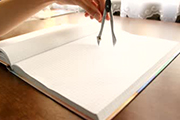What do you do when you realize a geometry problem has just popped up on the screen?

Try this GMATPrep problem from the free practice test and then well talk about what to do!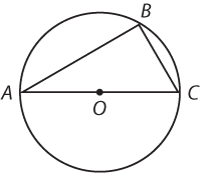* In the figure above, the radius of the circle with center O is 1 and BC = 1. What is the area of triangular region ABC?

(A) [pmath]sqrt{2}/2[/pmath]

(B)[pmath]sqrt{3}/2[/pmath]

(C) 1

(D)[pmath]sqrt{2}[/pmath]

(E) [pmath]sqrt{3}[/pmath]

Whats your first step? Lets use this problem as an opportunity to practice the Quant Process.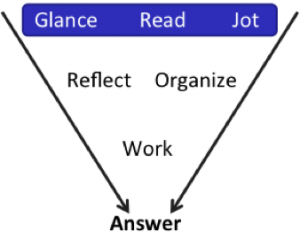At a glance, you can see that the problem provides a diagram. Draw! Make it big enough that you can add labels as you calculate new pieces of information (and, of course, jot down any information given in the problem).

Finally, write down any formulas youll need, as well as whatever the problem asks you to find. Your scrap paper might look something like this: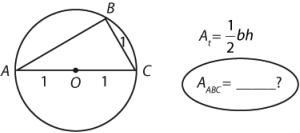The problem asks for the area of the triangle. You need a base and a height in order to calculate this. You know one possible base: AC = 2. If you draw a vertical line down from B to the base, that would be the corresponding height.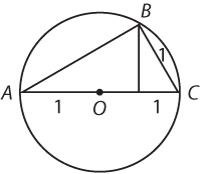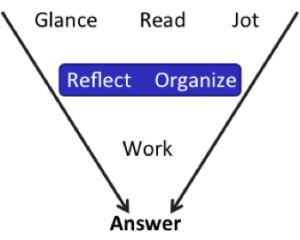Before you dive in and try to find this height, though, Reflect! Ask yourself whether there are other possible ways to move forward. Sometimes, the obvious way turns out not to be the easiest way to proceed.

In particular, this is a multi-shape problem: you were given both a triangle and a circle. Why did they include the circle? Pay particular attention to where the two shapes overlap.

Hmm. The hypotenuse of the triangle is also a diameter of the circle. How can you use that to solve?

It turns out that when a triangle is inscribed in a circle (the 3 vertices of the triangle all sit on the circle), and the hypotenuse of that triangle is also a diameter of the circle, then the triangle in question is a right triangle. (This is one of the rules were supposed to memorize for the test.)

In this case, the right angle is labeled B. Is that information useful at all? Well, if youre trying to find the area of a right triangle, then you just need to know the lengths of the two legs: AB and BC. The problem says that BC = 1, so the only unknown is AB.

Now you have a choice: do you think itll be easier to find the length of AB or to find the length of the vertical line that you drew in below point B?

Because ABC is a right triangle, its easier to find AB. The short leg is 1 and the hypotenuse is 2. Do those numbers match any of the smart triangles that youve studied? (If not, use the Pythagorean Theorem.)

Yes! These match the 30-60-90 triangle parameters.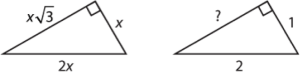The length of AB is [pmath]sqrt{3}[/pmath]. Plug this into the area formula:

[pmath]A={1/2}(sqrt{3})(1)[/pmath]

[pmath]A=sqrt{3}/2[/pmath]